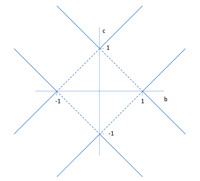## PT-Symmetric Representations of Fermionic Algebras

Carl M. Bender, S. P. KlevanskyA recent paper by Jones-Smith and Mathur extends PT-symmetric quantum mechanics from bosonic systems (systems for which $$T^2=1$$) to fermionic systems (systems for which $$T^2=-1$$). The current paper shows how the formalism developed by Jones-Smith and Mathur can be used to construct PT-symmetric matrix representations for operator algebras of the form $$\eta^2=0$$, $$\bar{\eta}^2=0$$, $$\eta\bar{\eta}+\bar {\eta} =\alpha 1$$, where $$\bar{eta}=\eta^{PT} =PT \eta T^{-1}P^{-1}$$. It is easy to construct matrix representations for the Grassmann algebra ($$\alpha=0$$). However, one can only construct matrix representations for the fermionic operator algebra ($$\alpha \neq 0$$) if $$\alpha= -1$$; a matrix representation does not exist for the conventional value $$\alpha=1$$.

http://arxiv.org/abs/1104.4156
Subjects: High Energy Physics – Theory (hep-th); Mathematical Physics (math-ph); Quantum Physics (quant-ph)